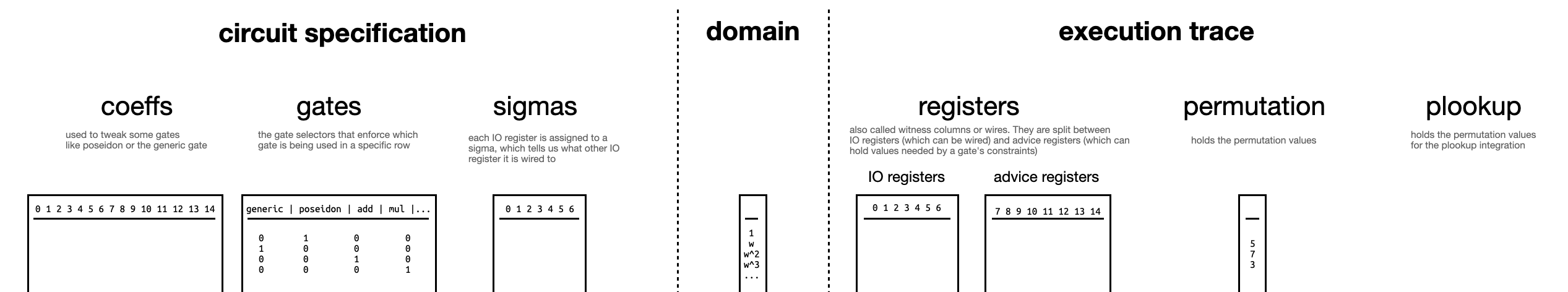# Arguments

In the previous section we saw how we can prove that certain equations hold for a given set of numbers very efficiently. What’s left to understand is the motivation behind these techniques. Why is it so important that we can perform these operations and what do these equations represent in the real world?

But first, let’s recall the table that summarizes some important notation that will be used extensively:One of the first ideas that we must grasp is the notion of a circuit. A circuit can be thought of as a set of gates with wires connections between them. The simplest type of circuit that one could think of is a boolean circuit. Boolean circuits only have binary values: and , true and false. From a very high level, boolean circuits are like an intricate network of pipes, and the values could be seen as water or no water. Then, gates will be like stopcocks, making water flow or not between the pipes. This video is a cool representation of this idea. Then, each of these behaviours will represent a gate (i.e. logic gates). One can have circuits that can perform more operations, for instance arithmetic circuits. Here, the type of gates available will be arithmetic operations (additions and multiplications) and wires could have numeric values and we could perform any arbitrary computation.

But if we loop the loop a bit more, we could come up with a single Generic gate that could represent any arithmetic operation at once. This thoughtful type of gate is the one gate whose concatenation is used in Plonk to describe any relation. Apart from it’s wires, these gates are tied to an array of coefficients that help describe the functionality. But the problem of this gate is that it takes a large set of them to represent any meaningful function. So instead, recent Plonk-like proof systems have appeared which use custom gates to represent repeatedly used functionalities more efficiently than as a series of generic gates connected to each other. Kimchi is one of these protocols. Currently, we give support to specific gates for the Poseidon hash function, the CompleteAdd operation for curve points, VarBaseMul for variable base multiplication, EndoMulScalar for endomorphism variable base scalar multiplication, RangeCheck for range checks and ForeignFieldMul and ForeignFieldAdd for foreign field arithmetic. Nonetheless, we have plans to further support many other gates soon, possibly even Cairo instructions.

The circuit structure is known ahead of time, as this is part of the public information that is shared with the verifier. What is secret is what we call the witness of the relation, which is the correct instantiation of the wires of the circuit satisfying all of the checks. This means the verifier knows what type of gate appears in each part of the circuit, and the coefficients that are attached to each of them.

The execution trace refers to the state of all the wires throughout the circuit, upon instantiation of the witness. It will be represented as a table where the rows correspond to each gate and the columns refer to the actual wires of the gate (a.k.a. input and output registers) and some auxiliary values needed for the computation (a.k.a. advice registers). The current implementation of Kimchi considers a total of 15 columns with the first 7 columns corresponding to I/O registers. Additionally, gates are allowed to access the elements in the current row Curr and the next Next. The permutation argument acts on the I/O registers (meaning, it will check that the cells in the first 7 columns of the execution trace will be copied correctly to their destination cells). For this reason, these checks are also known as copy constraints.

Going back to the main motivation of the scheme, recall that we wanted to check that certain equations hold in a given set of numbers. Here’s where this claim starts to make sense. The total number of rows in the execution trace will give us a domain. That is, we define a mapping between each of the row indices of the execution trace and the elements of a multiplicative group with as many elements as rows in the table. Two things to note here. First, if no such group exists we can pad with zeroes. Second, any multiplicative group has a generator whose powers generate the whole group. Then we can assign to each row a power of . Why do we want to do this? Because this will be the set over which we want to check our equations: we can transform a claim about the elements of a group to a claim like “these properties hold for each of the rows of this table”. Interesting, right?